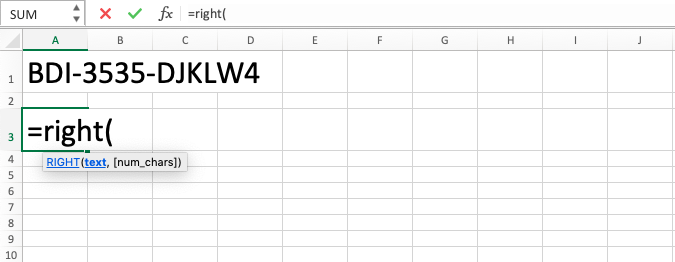RIGHT Function in Excel - Compute Expert

# RIGHT Function in Excel

In this tutorial, you will learn about RIGHT formula in excel. RIGHT function in excel can separate characters according to your needs from a data or cell content that is in this spreadsheet tool.

## Why We Need to Learn About RIGHT Formula in Excel?

If you have particular data or cell content which is a mix of some data that can be separated and part of that mix is your requirement for analysis process, then a way is needed to do the separation process. Sometimes, the thing which is wanted to be taken from the mix is at the most behind part of the data or cell content.

For example, imagine that you have a column in your spreadsheet with the full name content of your whole customers in an area. You want to take all of the last names from the column for email purposes. For that, you must take from behind all your full names to be grouped into another column with the header ‘Last Name’ that can be used for your email purpose.

If that full name column has much data, then it will be very tedious if you want to do the separation process manually.

Because of that, RIGHT function in excel is needed to solve the problem. This function can be used to take a part of characters from our data or cell content from the most behind position, just as we need for the previous case. Of course, RIGHT formula in excel can be used for another purpose if later you also need to take characters from the most behind position for your data analysis process.

## What is RIGHT Function in Excel?

RIGHT function in excel is a formula that can be used to take some characters according to your needs from a data or cell content with the starting position of the process from the most behind. In this RIGHT formula in excel, you need to input data or cell coordinate where the content will be taken from for the characters and also you need to input how many characters to be taken from the most behind position.

A brief explanation of the inputs in the formula is as follows:

=RIGHT(text, num_chars)

Note:
• text = the data that you want to take characters from
• num_chars = the amount of characters that you want to take from the most right position in the data

## How to Use RIGHT Formula in Excel?

The following will explain how to use RIGHT function in excel. Using RIGHT formula in excel is pretty easy and has similar writings if you have understood how to use LEFT formula.

## Using RIGHT Function in Excel

1. Type equal sign ( = ) in the cell where you want to put the result in2. Type RIGHT (can be with large and small letters) and open bracket sign after =3. Type data or cell coordinate where the data which you want to process with this formula is after open bracket sign followed by a comma sign ( , )4. Type the number to represent how many characters will you take from the most behind position/cell coordinate where the number is after comma sign5. Type close bracket sign6. Press Enter
7. The process is done!## Exercise

After you have learned how to use RIGHT formula in excel, you can practice your understanding through this exercise below!

### Questions

1. Take the year part from the question in column B!
2. Take the last name from the whole name of the person in column C!
3. Take the decimal number after the decimal separation point in column D!

There are other characters’ separation formulas in this spreadsheet software such as MID and LEFT. The difference with the formula explained here is in the starting position determination for the character separation from your data/cell content. If you want, then the tutorials can be learned also from other parts of this Compute Expert blog!

Get updated excel info from Compute Expert by registering your email. It's free!